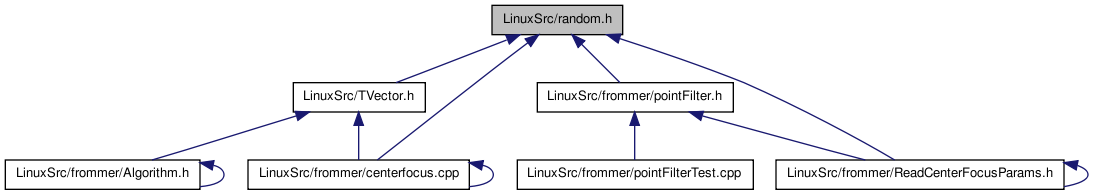# random.h File Reference

random number generator of L'Ecuyer with Bays-Durham shuffle and added safeguards (numeric recipes) More...

This graph shows which files directly or indirectly include this file:Go to the source code of this file.

## Defines

#define IM1   2147483563
#define IM2   2147483399
#define AM   (1.0/IM1)
#define IMM1   (IM1-1)
#define IA1   40014
#define IA2   40692
#define IQ1   53668
#define IQ2   52774
#define IR1   12211
#define IR2   3791
#define NTAB   32
#define NDIV   (1+IMM1/NTAB)
#define EPS   1.2e-7
#define RNMX   (1.0-EPS)
#define MODMULT(a, b, c, m, s)   q = s/a; s = b*(s-a*q)-c*q; if (s < 0) s += m;

## Functions

double ran2 (long *idum)
Long period (> 2.0e18) random number generator of L'Ecuyer with
Bays-Durham shuffle and added safeguards. Returns a uniform
random deviate between 0.0 and 1.0 (exclusive of the endpoints).
unsigned short random (long *seed, unsigned short max)
returns a random value between zero and max, Long period (> 2.0e18) (see ran2())
uint32_t randomUInt32 (long *seed, uint32_t max)
double combinedLCG (void)
void initLCG (long init_s1, long init_s2)

## Variables

static long CF_MM_s1 = 1
static long CF_MM_s2 = 1

## Detailed Description

Todo:
seed ist fuer die random-Funktion nicht dokumentiert.

Definition in file random.h.

## Define Documentation

 #define AM   (1.0/IM1)

Definition at line 79 of file random.h.

Referenced by ran2().

 #define EPS   1.2e-7

Definition at line 89 of file random.h.

 #define IA1   40014

Definition at line 81 of file random.h.

Referenced by ran2().

 #define IA2   40692

Definition at line 82 of file random.h.

Referenced by ran2().

 #define IM1   2147483563

Definition at line 77 of file random.h.

Referenced by ran2().

 #define IM2   2147483399

Definition at line 78 of file random.h.

Referenced by ran2().

 #define IMM1   (IM1-1)

Definition at line 80 of file random.h.

Referenced by ran2().

 #define IQ1   53668

Definition at line 83 of file random.h.

Referenced by ran2().

 #define IQ2   52774

Definition at line 84 of file random.h.

Referenced by ran2().

 #define IR1   12211

Definition at line 85 of file random.h.

Referenced by ran2().

 #define IR2   3791

Definition at line 86 of file random.h.

Referenced by ran2().

 #define MODMULT ( a, b, c, m, s ) q = s/a; s = b*(s-a*q)-c*q; if (s < 0) s += m;

Definition at line 256 of file random.h.

Referenced by combinedLCG().

 #define NDIV   (1+IMM1/NTAB)

Definition at line 88 of file random.h.

Referenced by ran2().

 #define NTAB   32

Definition at line 87 of file random.h.

Referenced by ran2().

 #define RNMX   (1.0-EPS)

Definition at line 90 of file random.h.

Referenced by ran2().

## Function Documentation

 double combinedLCG ( void )

Definition at line 258 of file random.h.

References CF_MM_s1, CF_MM_s2, MODMULT, and q().

Here is the call graph for this function:void initLCG ( long init_s1, long init_s2 )

Definition at line 270 of file random.h.

References CF_MM_s1, and CF_MM_s2.

 uint32_t randomUInt32 ( long * seed, uint32_t max ) ` [inline]`

Definition at line 214 of file random.h.

References ran2().

Here is the call graph for this function:## Variable Documentation

 long CF_MM_s1 = 1` [static]`

Definition at line 253 of file random.h.

Referenced by combinedLCG(), and initLCG().

 long CF_MM_s2 = 1` [static]`

Definition at line 254 of file random.h.

Referenced by combinedLCG(), and initLCG().

Generated on Tue Nov 23 13:10:52 2010 for centerfocus by1.6.3